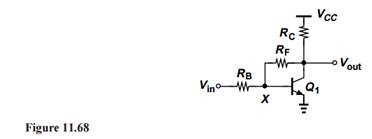### Create an Account

Home / Questions / Apply Miller’s theorem to resistor RF in Fig 11 68 and estimate the voltage gain of the c...

# Apply Miller’s theorem to resistor RF in Fig 11 68 and estimate the voltage gain of the circuit Assume VA ∞ and RF is large enough to allow the approximation

Apply Miller’s theorem to resistor RF in Fig. 11.68 and estimate the voltage gain of the circuit. Assume VA = ∞ and RF is large enough to allow the approximation vout = vX = gm RC.Apr 24 2020 View more View LessSubscribe To Get Solution